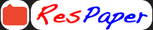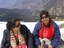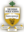Trending ▼   ResFinderICSE Prelims 2017 : Mathematics

6 pages, 1 questions, 0 questions with responses, 0 total responses,00Rashmi The Indian Public School, Dehradun
+Fave Message
 Home > rashmitaneja1 >Formatting page ...

THE INDIAN PUBLIC SCHOOL, DEHRADUN PRE-BOARD-I 2016-17 MATHEMATICS/CLASS X TIME: 2Hrs30Mins MM: 80 RST: 35 Answers to this paper must be written on the paper provided separately. You will not be allowed to write during first 15 minutes. This time is to be spent in reading the question paper. The time given at the head of this Paper is allowed for writing the answer. Answer all questions from section A and any four questions from section B. All working, including rough work, must be clearly shown and must be done on the same sheet as the rest of the answer. Omission of essential working will result in the loss of marks. The intended marks for questions or parts of questions are given in brackets [ ]. Section-A (40 Marks) Question 1. (a) On a certain sum of money, the difference between the compound interest for a year, payable half yearly, and the simple interest for a year is Rs. 128. Find the sum lent out, if the rate of interest in both case is 8%.  (b) Solve the following in equation and represent the solution set on the number line. 1 15 7 x >2 x 27 , x N  (c) A die has 6 faces marked by the given numbers as shown below:  1 2 3 -1 -2 -3 The die is thrown once. What is the probability of getting? (i) (ii) (iii) A positive integer. An integer greater than -3. The smallest integer. Question 2. (a) If A= [ 1 0 1 7 ] , then find the value of k, so that A =8A+kI. 2  (b) Sandhya has a 4 years recurring deposit account in State bank of India and deposits Rs.2500 per month. If she receives Rs.149400 at the time of maturity, find the rate of interest.  (c) (i) In the given figure, a right triangle BOA is given. C is the midpoint of the hypotenuse AB. Show that it is equidistance from the vertices O, A and B.Formatting page ...Formatting page ...Formatting page ...Formatting page ...Formatting page ...